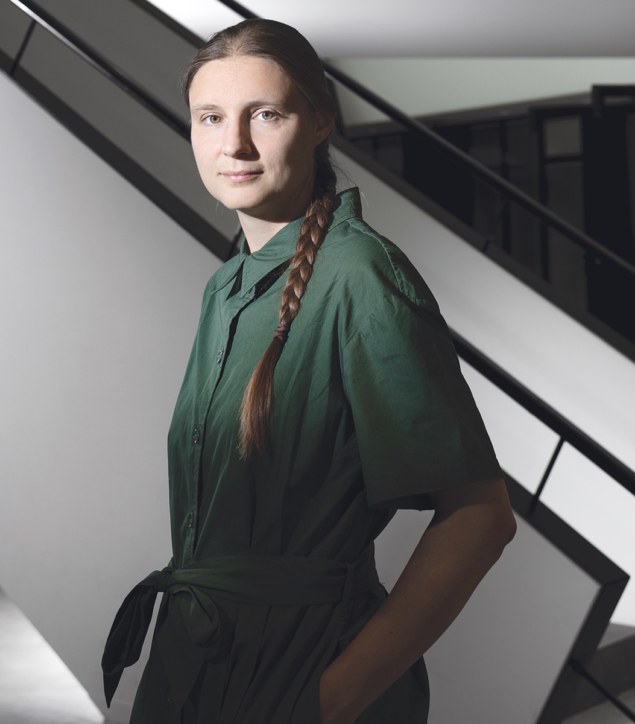# Playing in the sandbox of geometry

10 January 2023

Number theorist and Fields medallist Maryna Viazovska talks about her research, its applications to physics and the relationship between mathematics and reality.Higher spheres Ukrainian mathematician Maryna Viazovska is chair of number theory at EPFL, Lausanne, Switzerland. In 2022 she became the second woman in history to earn a Fields Medal, recognising her work on solving the sphere-packing problem. Credit: EPFL/F Merz

### When did you first know you had a passion for pure mathematics?

I have had a passion for mathematics since my first year in school. At that time I did not realise what “pure mathematics” was, but maths was my favourite subject from a very early age.

### What is number theory, in terms that a humble particle physicist can understand?

In fact, “number theory” is not well defined and any interesting question about numbers, geometric shapes and functions can be seen as a question for a number theorist.

### What motivated you to work on sphere-packing?

I think it is a beautiful problem, something that can be easily explained. Physicists know what a Euclidean space and a sphere are, and everybody knows the problem from stacking oranges or apples. What is a bit harder to explain is that mathematicians are not trying to model a particular physical situation. Mathematicians are not bound to phenomena in nature to justify their work, they just do it. We do not need to model any physical situation, which is a luxury. The work could have an accidental application, but this is not the primary goal. Physicists, especially theorists, are used to working in multi-dimensional spaces. At the same time, these dimensions have a special interpretation in physics.

### What fascinates you most about working on theoretical rather than applied mathematics?

My motivation often comes out of curiosity and my belief that the solutions to the problems will become useful at some point in the future. But it is not my job to judge or to define the usefulness. My belief is that the fundamental questions must be answered, so that other people can use this knowledge later. It is important to understand the phenomena in mathematics and in science in general, and the possibility of discovering something that other people have not yet. Maybe it is possible to come up with other ideas for detectors, which become interesting. When I look at physics detectors, for example, it fascinates me how complex these machines are and how many tiny technical solutions must be invented to make it all work.

### How did you go about cracking the sphere-stacking problem?

I think there was an element of luck that I could find the correct idea to solve this problem because many people worked on it before. I was fortunate to find the right solution. The initial problem came from geometry, but the final solution came from Fourier analysis, via a method called linear programming.

I think a mathematical reality exists on its own and sometimes it does describe actual physical phenomena

In 2003, mathematicians Henry Cohn and Noam Elkies applied the linear programming method to the sphere-packing problem and numerically obtained a nearly optimal upper bound in dimensions 8 and 24. Their method relied on constructing an auxiliary, “magic”, function. They computed this function numerically but could not find an explicit formula for it. My contribution was to find the explicit formula for the magic function.

### What applications does your work have, for example in quantum gravity?

After I solved the sphere-packing problem in dimension 8 in 2016, CERN physicists worked on the relation between two-dimensional conformal field theory and quantum gravity. From what I understand, conformal field theories are mathematically totally different from sphere-packing problems. However, if one wants to optimise certain parameters in the conformal field theory, physicists use a method called “bootstrap”, which is similar to the linear programming that I used. The magic functions I used to solve the sphere-packing problem were independently rediscovered by Thomas Hartman, Dalimil Mazác and Leonardo Rastelli.

### Are there applications beyond physics?

One of the founders of modern computer science, Claude Shannon, realised that sphere-packing problems are not only interesting geometric problems that pure mathematicians like me can play with, but they are also a good model for error-correcting codes, which is why higher-dimensional sphere packing problems became interesting for mathematicians. A very simplified version of the original model could be the following. An error is introduced during the transmission of a message. Assuming the error is under control, the corrupted message is still close to the original message. The remedy is to select different versions of the messages called codewords, which we think are close to the original message but at the same time far away from each other, so that they do not mix with each other. In geometric language, this situation is an exact analogy of sphere-packing, where each code word represents the centre of the sphere and the sphere around the centre represents the cloud of possible errors. The spheres will not intersect if their centres are far away from each other, which allows us to decode the corrupted message.

### Do you view mathematics as a tool, or a deeper property of reality?

Maybe it is a bit idealistic, but I think a mathematical reality exists on its own and sometimes it does describe actual physical phenomena, but it still deserves our attention if not. In our mathematical world, we have chances to realise that something from this abstract mathematical world is connected to other fields, such as physics, biology or computer science. Here I think it’s good to know that the laws of this abstract world often provide us with useful gadgets, which can be used later to describe the other realities. This whole process is a kind of “spiral of knowledge” and we are in one of its turns.